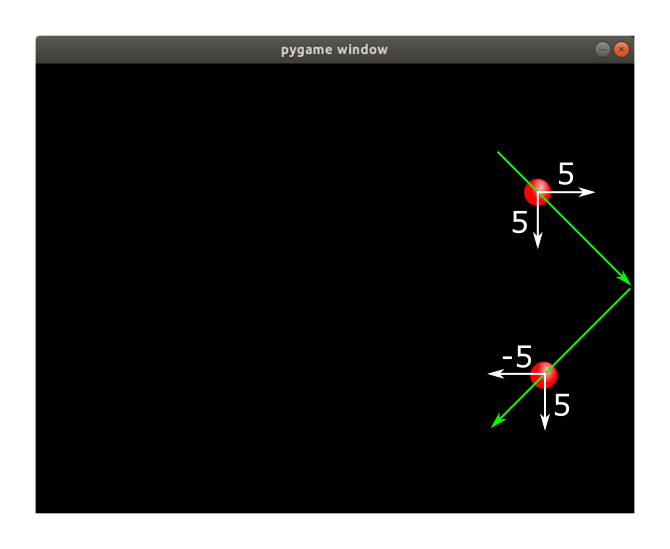# Bouncing ball in pygame

Martin McBride, 2021-03-09
Tags game development sprite animation
Categories pygameWe created a moving sprite in the previous article, but this time we will do something a bit more interesting. Rather than disappearing off the edge of the screen, we will make our sprite bounce off the edges.

## Modifying the sprite behaviour

We will make a couple of changes to the Ball class:

class Ball(pg.sprite.Sprite):

def __init__(self, pos):
super(Ball, self).__init__()
self.rect = self.image.get_rect()
self.rect.center = pos
self.velocity = [5, 5]

def update(self):
if self.rect.left < 0 or self.rect.right >= SCREEN_WIDTH:
self.velocity *= -1
if self.rect.top < 0 or self.rect.bottom >= SCREEN_HEIGHT:
self.velocity *= -1
self.rect.move_ip(self.velocity)


The first thing we have done is make the initial velocity [5, 5], so that the ball travels a bit faster.

But the main changes is in the update method. We test the position of the rectangle containing the ball, and if we are at the edge of the screen we reverse its velocity to make it start travelling in the opposite direction.

Specifically, if the left hand edge of the rectangle, rect.left, is less that zero, or if the right hand edge, rect.right, is greater than or equal to the screen width, then we know that the ball has hit the left or right edge of the screen. So we reverse the velocity of the x direction.

Here is an illustration of the ball approaching the right hand edge of the screen. It has a velocity of [5, 5], so it is travelling diagonally towards the bottom right. When it hits the edge, its x velocity is reversed, making its velocity [-5, 5]. This means it is travelling diagonally towards the bottom left:We do a similar check to see if the ball hits the top or bottom of the screen, and we reverse the velocity of the y direction if that happens.

It is possible that the ball could hit two sides at the same time. For example if it exactly hits the top left corner of the screen. That is no problem - the two if statements will both execute, and the ball will change direction in both x and y axes.

## Other changes

You might expect that we would need to modify the main loop to make our sprite bounce, but in fact we don't. The behaviour is totally contained within the Ball class.

The source code is available on github as bouncingsprite.py.

If you found this article useful, you might be interested in the book Computer Graphics in Python or other books by the same author.

#### Popular tags

2d arrays abstract data type alignment and angle animation arc array arrays bar chart bar style behavioural pattern bezier curve built-in function callable object chain circle classes clipping close closure cmyk colour combinations comparison operator comprehension context context manager conversion count creational pattern data science data types decorator design pattern device space dictionary drawing duck typing efficiency ellipse else encryption enumerate fill filter font font style for loop function function composition function plot functools game development generativepy tutorial generator geometry gif global variable gradient greyscale higher order function hsl html image image processing imagesurface immutable object in operator index inner function input installing iter iterable iterator itertools join l system lambda function len lerp line line plot line style linear gradient linspace list list comprehension logical operator lru_cache magic method mandelbrot mandelbrot set map marker style matplotlib monad mutability named parameter numeric python numpy object open operator optimisation optional parameter or pandas partial application path pattern permutations pie chart polygon positional parameter print pure function python standard library radial gradient range recipes rectangle recursion reduce regular polygon repeat rgb rotation roundrect scaling scatter plot scipy sector segment sequence setup shape singleton slice slicing sound spirograph sprite square str stream string stroke structural pattern subpath symmetric encryption template text text metrics tinkerbell fractal transform translation transparency triangle truthy value tuple turtle unpacking user space vectorisation webserver website while loop zip